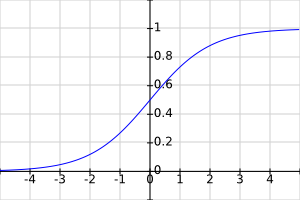# Conmajia

Stop stealing sheep!

## 前馈全连接神经网络和函数逼近、时间序列预测、手写数字识别

Andrew Kirillov 著
Conmajia 译
2019 年 1 月 12 日# 理论背景

## 人工神经元

$\tag{1} y=f\left(\sum_{j=1}^{m}\omega_j*x_j+b\right)$

$\tag{2} y=f(\mathbf{w}^\mathrm{T}\mathbf{x}+\mathbf{b})$

1943 年，沃伦·麦卡洛奇和沃尔特·皮茨提出了阈值逻辑单元（threshold logic unit），这是历史上首个实用的人工神经元. 它用一个阈值函数作为传递函数. 最初，只考虑了具有二进制输入/输出的简单模型，对模型可能的权重也只作了一些简单限制. 然而从一开始，人们就已经注意到任何布尔函数都可以通过这些设备的网络实现. 这一点可以从实现一个（AND）、（OR）函数看出.

## 和（AND）、或（OR）例子

$\mathrm{XOR}(x_1,x_2)=\mathrm{AND}(\mathrm{OR}(x_1,x_2),\mathrm{NAN}(x_1,x_2))$

## 人工神经网络

• $$l$$ 网络的层数
• $$n(k)$$$$k$$ 层神经元数量
• $$n(0)$$ 网络输入数量
• $$m(k)$$ 进入第 $$k$$ 层的输入数量（$$m(k)=n(k-1)$$
• $$y(k)$$$$k$$ 层输出的列向量，长度 $$n(k)$$
• $$y(0)$$ 网络输入的列向量（向量 $$\mathbf{x}$$
• $$b(k)$$$$k$$ 层偏差值的列向量，长度 $$n(k)$$
• $$\mathbf{w}(k)$$$$k$$ 层的权重矩阵. 矩阵的第 $$i$$ 行包含层的第 $$i$$ 个神经元的权重， $$n(k)\times m(k)$$

$\tag{3} y_{i}^{(k)}=f\left(\sum_{j=1}^{m^{(k)}}\omega_{i,j}^{(k)}*y_j^{(k-1)}+b_j^{(k)}\right),k=1,2,\cdots,l,i=1,2,\cdots,n^{(k)}$

$\tag{4} y_{i}^{(k)}=f\left(\mathbf{wy}^{(k-1)}+\mathbf{b}^{(k)}\right),k=1,2,\cdots,l$

## 激活函数

$f(x)=\frac{1}{1+e^{-x}}$

sigmoid 函数的形状类似于阶跃函数，如图 5-a，但没有那么尖锐，它是光滑的，可导的，连续的，值域 $$(0,1)$$. 但它并不完美，它也有它的问题. 尽管如此，它对于使用前馈全连接网络完成的不同分类任务依然能很好地工作，因此简便起见，还是继续使用它来进行研究.

f(x)=\left\{ \begin{aligned} 0 & , & x<0 \\ 1 & , & x\geqslant{0} \end{aligned} \right.图 5 sigmoid 函数和 $\tanh$ 函数图像

• 双曲正切，如图 5-b，形状类似于 sigmoid 函数，但值域是 $$(-1,1)$$
• SoftMax 函数，它将任意实值的向量压缩为实值的同一维向量，其中每个条目都在 $$(0,1)$$ 范围内，所有条目和为 1. 这有利于处理分类任务. 在分类任务中，神经网络的输出视为属于某个类的概率，概率之和恒为 1

## 成本函数

$\mathrm{Cost}_1=\frac{1}{2n}\sum_{i=1}^{n}\left(y_i-t_i\right)^2$

\tag{5} \begin{align*} \mathrm{Cost}&=\frac{1}{m}\sum_{j=1}^{m}\mathrm{Cost}_j\\ &=\frac{1}{2nm}\sum_{j=1}^{m}\sum_{i=1}^{n}\left(y_i^{(j)}-t_i^{(j)}\right)^2 \end{align*}

## 随机梯度下降算法

$\tag{6} \mathbf{a}^{(n+1)}=\mathbf{a}^{(n)}-\lambda\nabla \mathbf{F}\left(\mathbf{a}^{(n)}\right)$

$$\lambda$$ 足够小，$$\mathbf{F}\left(\mathbf{a}^{(n+1)}\right)\leqslant\mathbf{F}\left(\mathbf{a}^{(n)}\right)$$. 在函数 $$\mathbf{F}$$ 的某些假设下，可以保证收敛到局部极小值.

$\mathbf{\omega}^{(n+1)}=\mathbf{\omega}^{(n)}-\lambda\nabla\mathrm{Cost}\left(\mathbf{\omega}^{(n)}\right)$

$\tag{7} \mathbf{\omega}^{(n+1)}=\mathbf{\omega}^{(n)}-\lambda\nabla\mathrm{Cost}_j\left(\mathbf{\omega}^{(n)}\right)$

## 梯度和链式法则

$\tag{8} \omega_{i,j}^{(n+1)}=\omega_{i,j}^{(n)}-\lambda\frac{\partial\mathrm{Cost}_1}{\partial\omega_{i,j}^{(n)}}$

$\frac{\partial f}{\partial a}=\frac{\partial f}{\partial x}\frac{\partial x}{\partial t}\frac{\partial t}{\partial a}$

$\tag{9} \frac{\partial \mathrm{Cost}_1}{\partial \omega_{i,j}}=\frac{\partial \mathrm{Cost}_1}{\partial y_i}\frac{\partial y_i}{\partial u_i}\frac{\partial u_i}{\partial \omega_{i,j}}$

\tag{10} \begin{align*} \frac{\partial \mathrm{Cost}_1}{\partial y_i}&=\frac{\partial}{\partial y_i}\left[\frac{1}{2}\sum_{i=1}^{n}(y_i-t_i)^2\right] \\ &=\frac{\partial}{\partial y_i}\left[\frac{1}{2}(y_i-t_i)^2\right] \\ &=y_i-t_i \end{align*}

\begin{align*} \frac{\partial y_i}{\partial u_i}&=\frac{\partial}{\partial u_i}\frac{1}{1+e^{-u_i}} \\ &=\frac{e^{-u_i}}{(1+e^{-u_i})^2}\\ &=y_i(1-y_i) \end{align*}

sigmoid 函数的导数可以用两种方式定义. 其中之一是基于 $u_i$. 但是在人工神经网络中鲜少如此. 用函数本身的值来计算 sigmoid 的导数要快得多.

\begin{align*} \frac{\partial u_i}{\partial \omega_{i,j}}&=\frac{\partial}{\partial \omega_{i,j}}\left(\sum_{j=1}^{m}\omega_{i,j}*x_j+b_i\right) \\ &=\frac{\partial}{\partial \omega_{i,j}}\left(\omega_{i,j}*x_j\right) \\ &=x_j \end{align*}

\begin{align*} \frac{\partial u_i}{\partial b_i} &=\frac{\partial}{\partial b_i}\left(\sum_{j=1}^{m}\omega_{i,j}*x_j+b_i\right) \\ &=\frac{\partial b_i}{\partial b_i} \\ &=1 \end{align*}

\begin{align*} \omega_{i,j}^{(n+1)} &= \omega_{i,j}^{(n)}-\lambda\left[(y_i-t_i)y_i(1-y_i)x_j\right] \\ b_i^{(n+1)} &=b_i^{(n)}-\lambda\left[(y_i-t_i)y_i(1-y_i)\right] \end{align*}

## 误差反向传播

$\tag{10'} E_i=\frac{\partial\mathrm{Cost}_1}{\partial y_i}=y_i-t_i$

\begin{align*} E'_j&=\frac{\partial\mathrm{Cost}_1}{\partial y_1}\frac{\partial y_1}{\partial u_1}\frac{\partial u_1}{\partial y'_j}+\frac{\partial\mathrm{Cost}_1}{\partial y_2}\frac{\partial y_2}{\partial u_2}\frac{\partial u_2}{\partial y'_j}+\cdots+\frac{\partial\mathrm{Cost}_1}{\partial y_n}\frac{\partial y_n}{\partial u_n}\frac{\partial u_n}{\partial y'_j} \\ &=\sum_{i=1}^{n}\frac{\partial\mathrm{Cost}_1}{\partial y_i}\frac{\partial y_i}{\partial u_i}\frac{\partial u_i}{\partial y'_j} \end{align*}

\tag{11} \begin{align*} E'_j&=\sum_{i=1}^{n}E_i\frac{\partial y_i}{\partial u_i}\frac{\partial u_i}{\partial x_j}\\ &=\sum_{i=1}^{n}E_iy_i(1-y_i)\omega_{i,j} \end{align*}

\begin{align*} \omega_{i,j}^{(n+1)}&=\omega_{i,j}^{(n)}-\lambda\left[E_iy_i(1-y_i)x_j\right] \\ b_{i}^{(n+1)}&=b_i^{(n)}-\lambda\left[E_iy_i(1-y_i)\right] \end{align*}

# ANNT 库

ANNT 的类关系图如图 6：

XNeuralNetwork 类表示实际的神经网络. 网络的体系结构取决于放入其中的层的类型. 本文只介绍了前馈完全连接的神经网络例子. 在下一篇文章中，我将探讨卷积神经网络和循环神经网络.

# 使用范例

## 函数逼近

// 准备两层全连接人工神经网络
shared_ptr<XNeuralNetwork> net = make_shared<XNeuralNetwork>( );

net->AddLayer( make_shared<XFullyConnectedLayer>( 1, 10 ) ); // 1 输入, 10 神经元
net->AddLayer( make_shared<XFullyConnectedLayer>( 10, 1 ) ); // 10 输入, 1 神经元


// 用 Nesterov 优化器和 MSE 成本函数生成训练内容
XNetworkTraining netTraining( net,
make_shared<XNesterovMomentumOptimizer>( ),
make_shared<XMSECost>( ) );


for ( size_t epoch = 1; epoch <= trainingParams.EpochsCount; epoch++ )
{
// 打乱顺序
for ( size_t i = 0; i < samplesCount / 2; i++ )
{
int swapIndex1 = rand( ) % samplesCount;
int swapIndex2 = rand( ) % samplesCount;

std::swap( ptrInputs[swapIndex1], ptrInputs[swapIndex2] );
std::swap( ptrTargetOutputs[swapIndex1], ptrTargetOutputs[swapIndex2] );
}

auto cost = netTraining.TrainEpoch( ptrInputs, ptrTargetOutputs, trainingParams.BatchSize );
}


## 时间序列预测

// 准备 2 层人工神经网络，5 输入 1 输出 10 隐藏神经元
shared_ptr<XNeuralNetwork> net = make_shared<XNeuralNetwork>( );

net->AddLayer( make_shared<XFullyConnectedLayer>( 5, 10 ) );
net->AddLayer( make_shared<XFullyConnectedLayer>( 10, 1 ) );

// 用 Nesterov 优化器和 MSE 成本函数生成训练内容
XNetworkTraining netTraining( net,
make_shared<XNesterovMomentumOptimizer>( ),
make_shared<XMSECost>( ) );

for ( size_t epoch = 1; epoch <= trainingParams.EpochsCount; epoch++ )
{
// 打乱顺序
for ( size_t i = 0; i < samplesCount / 2; i++ )
{
int swapIndex1 = rand( ) % samplesCount;
int swapIndex2 = rand( ) % samplesCount;

std::swap( ptrInputs[swapIndex1], ptrInputs[swapIndex2] );
std::swap( ptrTargetOutputs[swapIndex1], ptrTargetOutputs[swapIndex2] );
}

auto cost = netTraining.TrainEpoch( ptrInputs, ptrTargetOutputs, trainingParams.BatchSize );
}


## 异或函数的二进制分类

// 准备 XOR 训练数据，输入编码为 -1、1，输出编码为 0、1
vector<fvector_t> inputs;
vector<fvector_t> targetOutputs;

inputs.push_back( { -1.0f, -1.0f } ); /* -> */ targetOutputs.push_back( { 0.0f } );
inputs.push_back( {  1.0f, -1.0f } ); /* -> */ targetOutputs.push_back( { 1.0f } );
inputs.push_back( { -1.0f,  1.0f } ); /* -> */ targetOutputs.push_back( { 1.0f } );
inputs.push_back( {  1.0f,  1.0f } ); /* -> */ targetOutputs.push_back( { 0.0f } );

// 准备 2 层人工神经网络
// 对 AND、OR 函数而言，单层就足够了，但是 XOR 需要两层，这点在前面讨论过
shared_ptr<XNeuralNetwork> net = make_shared<XNeuralNetwork>( );

net->AddLayer( make_shared<XFullyConnectedLayer>( 2, 2 ) );
net->AddLayer( make_shared<XFullyConnectedLayer>( 2, 1 ) );

// 用 Nesterov 优化器和二进制交叉熵成本函数生成训练内容
XNetworkTraining netTraining( net,
make_shared<XMomentumOptimizer>( 0.1f ),
make_shared<XBinaryCrossEntropyCost>( ) );

// 训练神经网络
printf( "每个样本的成本: \n" );
for ( size_t i = 0; i < 80 * 2; i++ )
{
size_t sample = rand( ) % inputs.size( );
auto   cost   = netTraining.TrainSample( inputs[sample], targetOutputs[sample] );
}


全连接人工神经网络 XOR 分类例程

{ -1.00 -1.00 } -> {  0.54 }
{  1.00 -1.00 } -> {  0.47 }
{ -1.00  1.00 } -> {  0.53 }
{  1.00  1.00 } -> {  0.46 }

0.6262 0.5716 0.4806 1.0270 0.8960 0.8489 0.7270 0.9774
...
0.0260 0.0164 0.0251 0.0161 0.0198 0.0199 0.0191 0.0152

{ -1.00 -1.00 } -> {  0.02 }
{  1.00 -1.00 } -> {  0.98 }
{ -1.00  1.00 } -> {  0.98 }
{  1.00  1.00 } -> {  0.01 }


## 鸢尾花多类分类

// 准备 3 层人工神经网络
shared_ptr<XNeuralNetwork> net = make_shared<XNeuralNetwork>( );

net->AddLayer( make_shared<XFullyConnectedLayer>( 4, 10 ) );
net->AddLayer( make_shared<XFullyConnectedLayer>( 10, 10 ) );
net->AddLayer( make_shared<XFullyConnectedLayer>( 10, 3 ) );

// 用 Nesterov 优化器和交叉熵成本函数生成训练内容
shared_ptr<XNetworkTraining> netTraining = make_shared<XNetworkTraining>( net,
make_shared<XNesterovMomentumOptimizer>( 0.01f ),
make_shared<XCrossEntropyCost>( ) );

// 用助手类训练人工神经网络分类
XClassificationTrainingHelper trainingHelper( netTraining, argc, argv );
trainingHelper.SetTestSamples( testAttributes, encodedTestLabels, testLabels );

// 40 世代, 每批 10 样本
trainingHelper.RunTraining( 40, 10, trainAttributes, encodedTrainLabels, trainLabels );


## MNIST 手写数字分类

// 准备 3 层人工神经网络
shared_ptr<XNeuralNetwork> net = make_shared<XNeuralNetwork>( );

net->AddLayer( make_shared<XFullyConnectedLayer>( trainImages.size( ), 300 ) );
net->AddLayer( make_shared<XFullyConnectedLayer>( 300, 100 ) );
net->AddLayer( make_shared<XFullyConnectedLayer>( 100, 10 ) );

// 用 Nesterov 优化器和交叉熵成本函数生成训练内容
shared_ptr<XNetworkTraining> netTraining = make_shared<XNetworkTraining>( net,
make_shared<XCrossEntropyCost>( ) );

// 用助手类训练人工神经网络分类
XClassificationTrainingHelper trainingHelper( netTraining, argc, argv );
trainingHelper.SetValidationSamples( validationImages, encodedValidationLabels, validationLabels );
trainingHelper.SetTestSamples( testImages, encodedTestLabels, testLabels );

// 20 世代, 每批 50 样本
trainingHelper.RunTraining( 20, 50, trainImages, encodedTrainLabels, trainLabels );


# 关于作者Andrew Kirillov，来自英国🇬🇧，目前就职于 IBM.

1. 过去研究内容相对较少，学习可以集中在课题本身. 现在各种衍生变化让人眼花撩乱，加之太多乌合之众也参与到“科技前沿”中来，鱼目混珠，难免令人困惑. ↩︎

2. 出自 Michael J. Garbade 博士 How to Create a Simple Neural Network in Python. ↩︎

3. 实际上这是第二篇，第一篇是介绍 ANNT 库结构和基本功能的文章. 感兴趣的读者可以参考这篇译文. ↩︎

4. Warren Sturgis McCulloch（1898-1969），美国神经生理学家和控制论学家. ↩︎

5. Walter Harry Pitts Jr.（1923-1969），美国逻辑学家. ↩︎

6. 最简单的阶跃函数也叫开关函数，表达式为： ↩︎

7. $$f(x)=x^+=max(0,x)$$，即输出为输入的正数部分. ↩︎

8. 我认为使用作为 epoch 的翻译更贴切. 相比之下，迭代（iteration）这个词太肤浅，没有体现出经由某一过程进化改变的精髓. 即便如此，很多人还是用迭代用得乐此不疲，也是无所谓，开心就好. ↩︎

9. 这是一个典型的策略模式（strategy pattern）设计. ↩︎

10. 一种高度优化的多线程并行编译处理方案. ↩︎

posted on 2019-01-15 11:25  Conmajia  阅读(2982)  评论(0编辑  收藏  举报